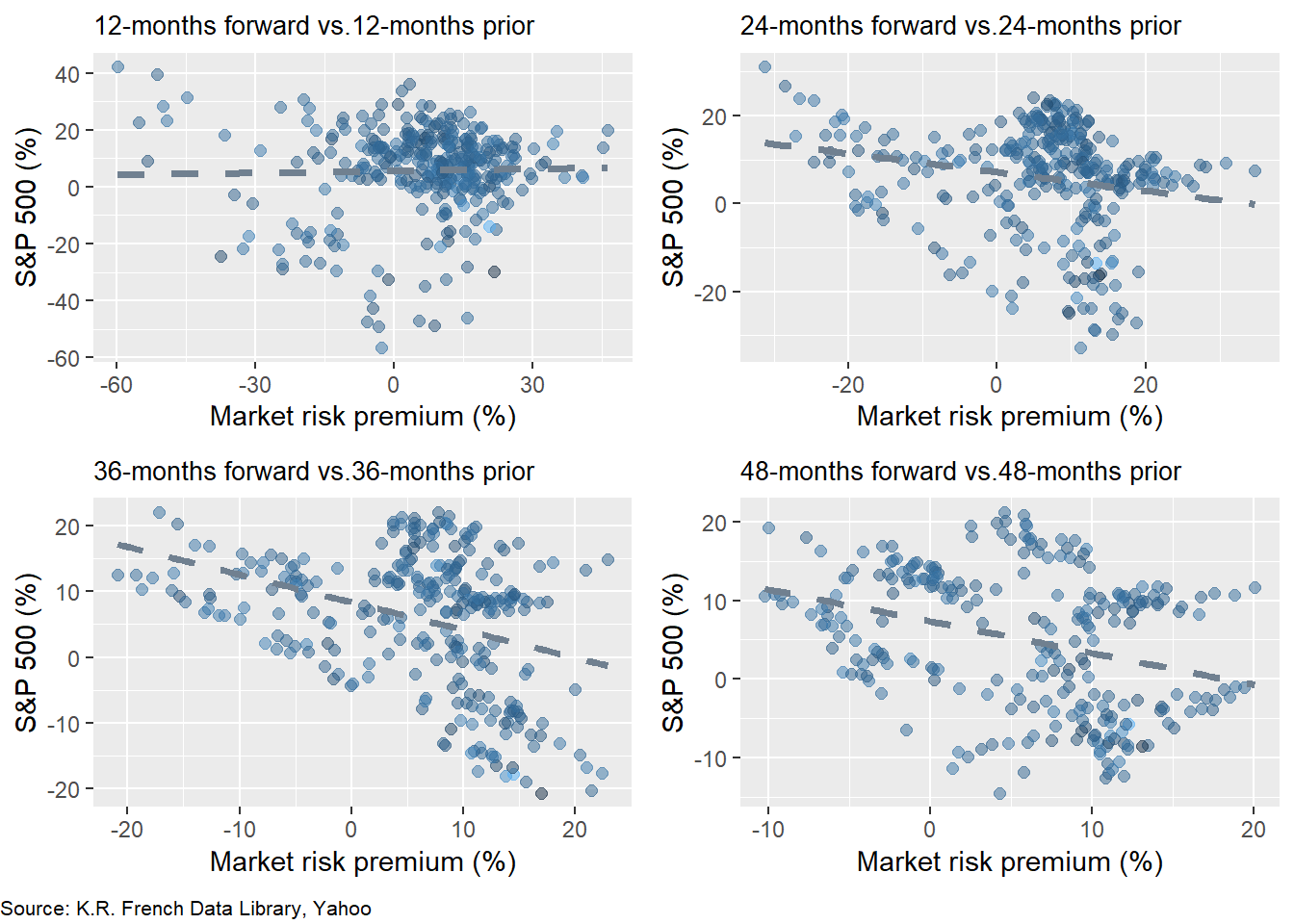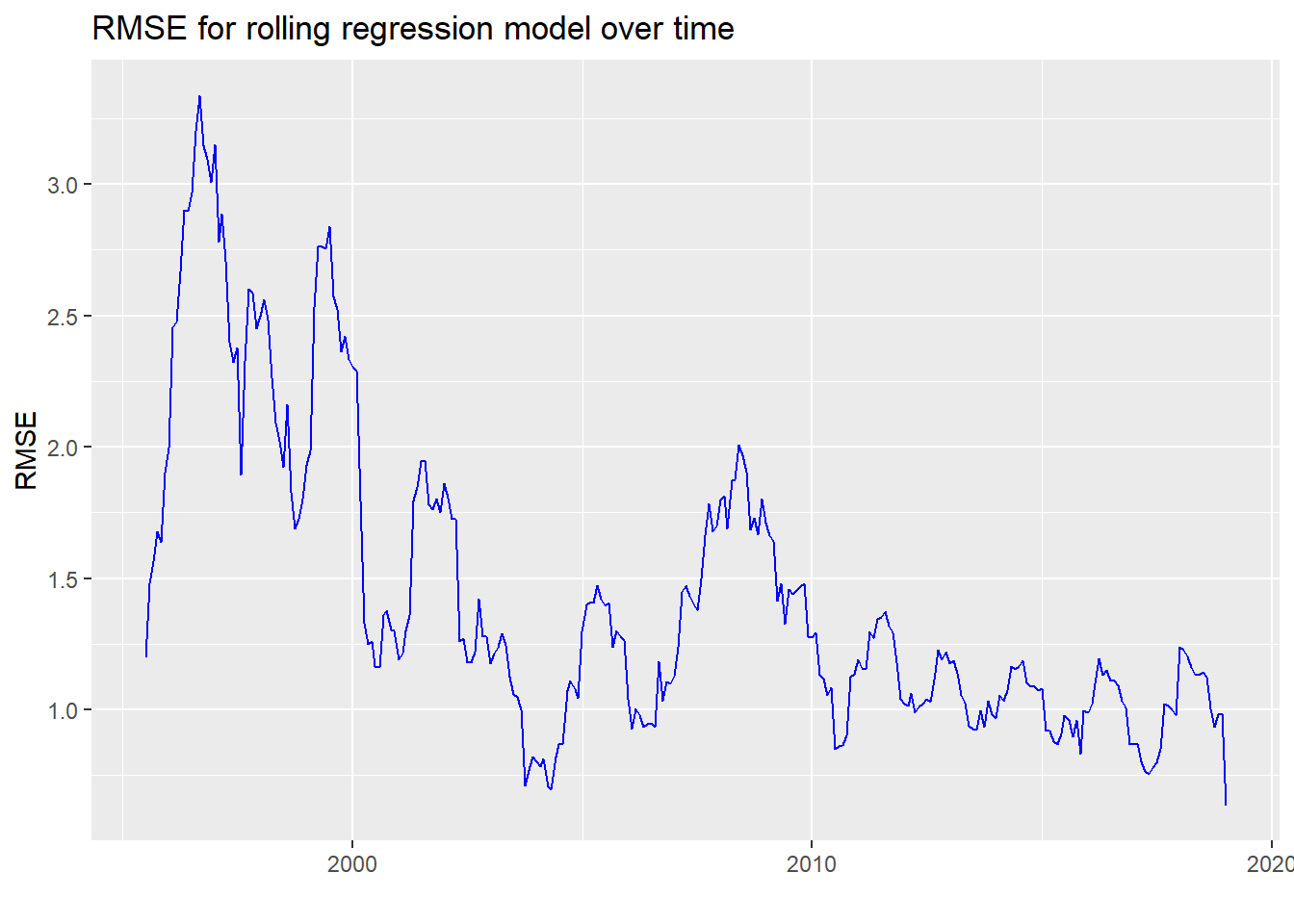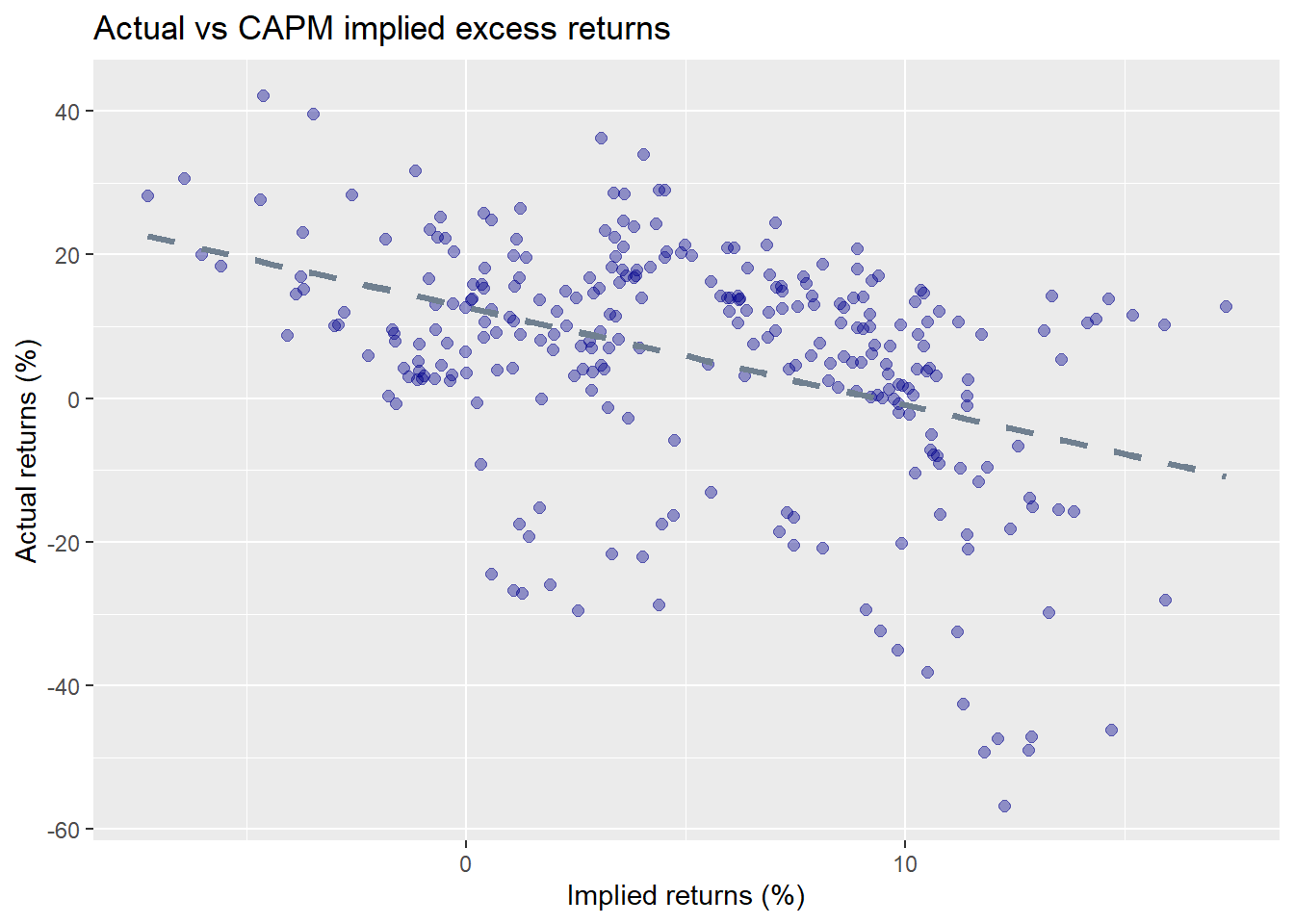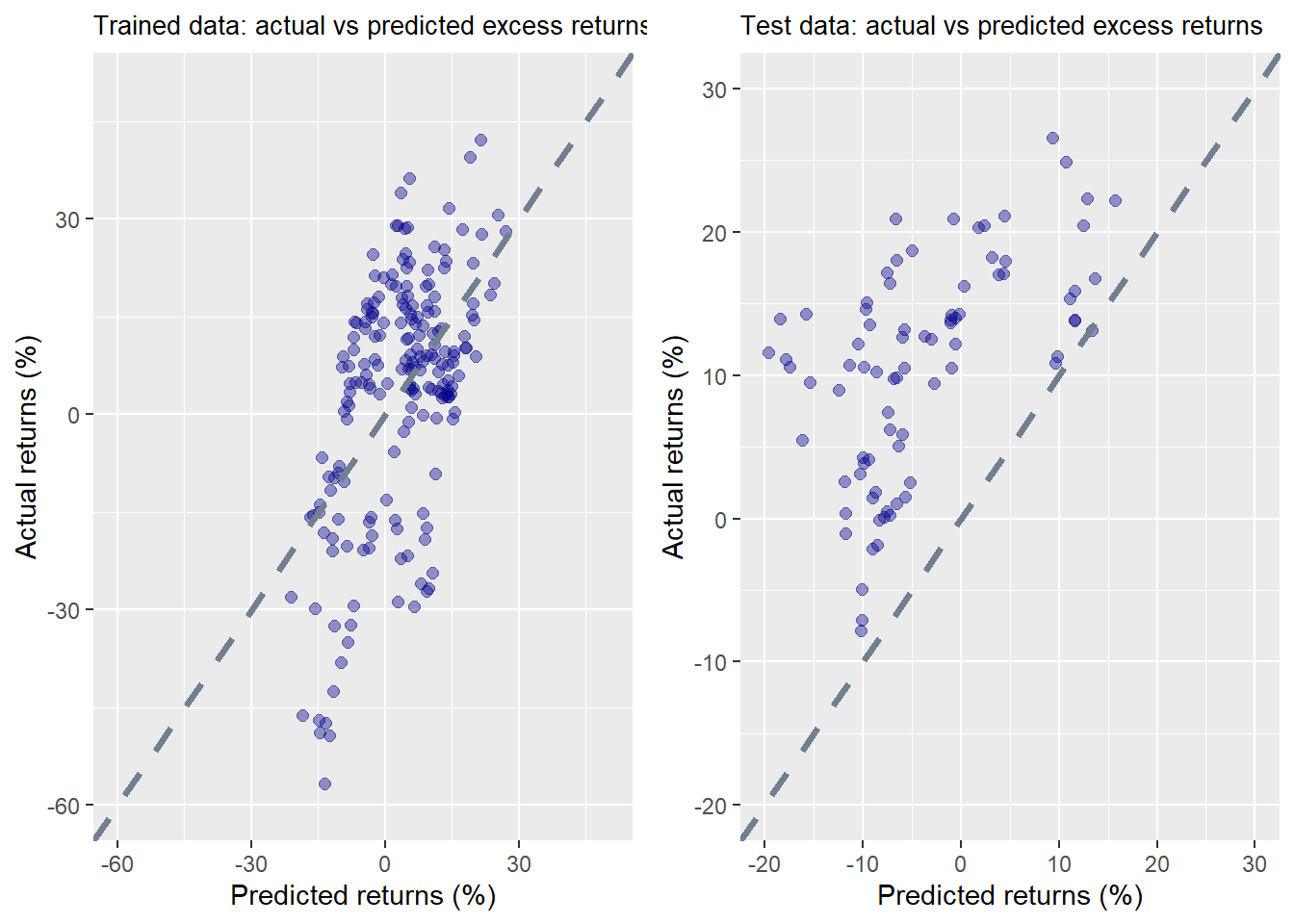# Machined risk premia

Over the last few posts, we’ve discussed methods to set return expectations to construct a satisfactory portfolio. These methods are historical averages, discounted cash flow models, and risk premia. our last post, focused on the third method: risk premia. Using the Capital Asset Pricing Model (CAPM) one can derive the required return for a particular asset based on the market price of risk, the asset’s risk, and the asset’s correlation with the market. While this model is elegant and intuitive, using it to forecast future returns didn’t produce the greatest results. Indeed one model based on CAPM yielded a false positive rate in excess of 80%. And so, we left the last post at a crossroads: examine risk premia more or move on to analyze which return expectation setting method was the most suitable. Based on the overwhelming responses received, we decided to examine risk premia further.

Given the poor forecasting results, Is CAPM a bad model? We don’t think so. We mustn’t forget that there are at least two types of models: descriptive or predictive, CAPM doesn’t purport to be predictive. At a minimum, its descriptive. It might even be normative, but that’s a discussion for another time.

Why didn’t the CAPM produce a reasonable forecast of future returns? The main reason is that there isn’t much of relationship between past risk premia and future returns. In the graph below, we average the prior period annualized monthly market risk premium and then compare it to excess return for the S&P 500 for the same period length in the future. For example, if we average the prior 12 months of monthly risk premia, we compare it to the next twelve months of excess returns.The scatter plots with lead-lag returns of 12 and 24 months show almost no linear relationship. Yet the scatter plots of 36 and 48 months exhibit a negative relationship with a good deal of noise. These results warrant a few comments as well as begs a question.

Just like so many investment offerings, the warning “past performance is not indicative of future results” bears itself out in these charts. What the market risk premium has been over the past months seems to have little bearing on what on future returns will be. That makes sense given CAPM’s descriptive nature: the model identifies the market clearing price of risk today, not tomorrow or many days after tomorrow. But negative returns over a longer time frame?

There are a couple interconnected parts to explaining this phenomenon. First, there is some mean reversion, so as the risk premium gets too far away from its average, future returns get pulled back. Second, the risk premium figure may not be the “true” risk premium. Recall, that the market risk premium is defined as the return in excess of the risk-free rate. But even though market returns exceed risk-free returns, that difference may not be entirely due to risk. Other factors like momentum, behavior, or central bank policy may influence this excess return. The presence of these other factors confound the relationship between past risk and future returns.1

That begs the question whether all the CAPM is measuring is the market’s relationship with itself since returns on most risky assets contribute to the overall market return. But this criticism misses the point that one should still be compensated for taking risk by receiving an excess return. Whether or not the excess return is commensurate with the risk is beyond the scope of this post. Nonetheless, if we accept that returns are mean-reverting and that excess returns capture some unobserved risk premium plus noise, then it makes sense that the longer time period average risk premia exhibit a negative relationship with future returns. Extreme moves will pull up the averages that will then correct themselves in the future.

One might agree or not with the foregoing explanation. Either way, it doesn’t help us with setting expected returns. Using a poor forecasting tool seems like a lesson futiliy.2 Yet, if a model is consistently wrong in the same way, doing the opposite might prove to be a viable strategy!

What’s plan? We need to figure out if cutting the risk premia data (without killing it!) in a different way can say anything about future returns. To do this, we’ll run a rolling 60-month regression of the S&P 500’s return vs. the market risk premium. Using that model, we’ll forecast the next twelve months of returns and then calculate the root mean-squared error (RMSE). We graph the RMSE over time below.We notice that the error rate really peaks prior to the tech bubble and then at the global financial crisis. While we end the data with 2019, we’d no doubt see similar peaks in 2020. The average RMSE is 1.44 while the scaled RMSE (RMSE over the average of the actuals) is 0.94, which tells you that average error is almost the same as the average return. While this might seem pretty bad, recall we were getting a scaled error rate of close to 2 in out bootstrap model from the prior post. Perhaps a rolling regression offers some degree hope.

How can we use these results? We see apart from the couple of spikes, the error rate has been relatively consistent in the 0.75 to 1.25 range. This consistency might be exploited in a machine learning model. We’ll see if we can generate a relationship between what a backward-looking CAPM implies for future excess returns and what actually happens. We’ll train the model by regressing actual returns on expected returns and then test it on out-of-sample data. First, we graph the actual vs. implied next twelve month average annualized return based on a rolling 60-month CAPM to get a sense of the data. We’ll call this the naive model.As expected, there is a negative relationship between implied and actual excess returns and the graph doesn’t look too different from ones above. The RMSE for this series is 19.2 with the scaled RMSE at 3.4. Pretty atrocious.

Let’s see if a machine can learn from this error to build a better model. We’ll train the model using about 70% of the data and then test on the remaining 30%. We show the scatter plots of the actual vs predicted returns on the training and test sets below. We also scale the axes equally and include a 45o line to show the divergences better.The results on trained data don’t look too bad. But the test results are a bit of a head scratcher. In every case, actual returns turned out to be higher than predicted. While we’ve rarely seen such a result, it’s easily explained by the time period, which ran from the end of 2012 to 2019, an extended bull market.

We present the RMSE and scaled RMSE below. In both the training set and the test, the RMSE is better than the RMSE from the naive model above. So this machine learning model does improve upon simply using the previous period’s risk premium to set future expected returns.

Table 1: Machine learning error results
Data RMSE Scaled RMSE
Train 15.7 4.6
Test 17.1 1.6

Interestingly, even though the RMSE is worse for the test set, it’s scaled RMSE is much better. It’s also almost two times better than the actual vs. predicted error rate of the naive model.

Before we start high-fiving, let’s figure out why the scaled RMSE on the test set is so much better. Even though the RMSE on the test is higher, the average return of the test set actuals at 10.6% is over three times the average return of the training set actuals at 3.4%. So it wasn’t the model, but an artifact of the actual data that made scaled RMSE better.

In the end, even though we built a better model, it wasn’t that much better; luck helped too, Moreover, it was somewhat convoluted, more art than science, and not immediately intuitive. The most important outcome of this exercise, then, is not so much the accuracy or error rates, but what it tells us about understanding risk premia. Last period’s risk premium isn’t that great at predicting future excess returns, even after using machine learning to adjust for the tendency toward error. Presumably, if we knew what risk premia would be in the future, we’d be better at building a model of future excess returns. Since we don’t have a crystal ball, we need to take a different approach. We could try to forecast future risk premia or we could derive the future implied risk premium based on market expectations and then compare that to actual results. The first approach could use some moving average or GARCH model. But this will have to wait since those methods would take us a bit more afield. The second method uses a discounted cash flow model to derive risk premia, which we’ll explain in our next post. Until then, here is the code behind all the models and graphs.

## Load packages
suppressPackageStartupMessages({
library(tidyquant)
library(tidyverse)
library(broom)
library(grid)
})

sp <- getSymbols("^GSPC", src="yahoo",
from="1950-01-01",
to="2020-01-01",
auto.assign = FALSE) %>%
colnames<-("price")

ff_url <- "http://mba.tuck.dartmouth.edu/pages/faculty/ken.french/ftp/Developed_3_Factors_CSV.zip"

GET(ff_url, write_disk(tf1 <- tempfile(fileext = ".zip")))
rename("date" = X1) %>%
mutate(date = ymd(parse_date_time(date, "%Y%m"))) %>%
na.omit()

## Convert S&_ to monthly
sp_mon <- to.monthly(sp, indexAt = "lastof", OHLC = FALSE)
sp_mon_df <- data.frame(date = index(sp_mon), price = as.numeric(sp_mon)) %>%
filter(date >="1990-07-01")

f_f <- f_f %>%
filter(date <= "2019-12-31" ) %>%
mutate(date = sp_mon_df$date) %>% mutate_at(vars(-"date"), as.numeric) ## Merge data sp_df <- sp_mon_df %>% left_join(f_f, "date") %>% mutate(ret = 100*(price/lag(price)-1), x_ret = ret - RF) %>% rename("Mkt" = "Mkt-RF") ## EDA lead_lag_plot <- function(lag_pd, lead_pd, method = "lm"){ sp_df %>% mutate(for_ret = lead(x_ret, lead_pd)) %>% na.omit() %>% mutate(mkt_mean = runMean(Mkt,lag_pd)*12, for_mean = runMean(for_ret, lead_pd)*12) %>% ggplot(aes(mkt_mean, for_mean)) + geom_point(aes(color=SMB), size=3, alpha=0.5) + geom_smooth(method = method, se=FALSE, color = "slategrey", size = 1.25, linetype="dashed") + labs(x="Market risk premium (%)", y="S&P 500 (%)", title = paste(lead_pd,"-months forward vs.", lag_pd, "-months prior", sep="")) + theme(legend.position = "") } p_12 <- lead_lag_plot(12,12) p_24 <- lead_lag_plot(24,24) p_36 <- lead_lag_plot(36,36) p_48 <- lead_lag_plot(48,48) gridExtra::grid.arrange(p_12, p_24, p_36, p_48, nrow=2, bottom = grid.text("Source: K.R. French Data Library, Yahoo", just = "left", x=0, y=0.5, gp = gpar(fontsize=2))) ### Rolling linear regression 60 months prior vs 12 months forward tests <- c() test_scaled <- c() for(i in 60:(nrow(sp_df)-12)){ y_dat <- sp_df$x_ret[(i-59):i]
x_dat <- sp_df$Mkt[(i-59):i] model <- lm(y_dat ~ x_dat) preds <- predict(model, data.frame(x_dat = sp_df$Mkt[(i+1):(i+12)]))
actuals <- sp_df$x_ret[(i+1):(i+12)] rmse <- sqrt(mean((preds-actuals)^2)) tests[i-59] <- rmse test_scaled[i-59] <- rmse/mean(actuals) } mean_rmse <- mean(tests, na.rm = TRUE) mean_scaled_rmse <- mean(test_scaled, na.rm = TRUE) ggplot() + geom_line(aes(sp_df$date[60:(nrow(sp_df)-12)], tests),
color = "blue") +
labs(x="",
y = "RMSE",
title = "RMSE by date for rolling regression model")

### Create data set of rolling figures
start <- 60
preds <- c()
actuals <- c()

for(i in start:(nrow(sp_df)-12)){
y_dat <- sp_df$x_ret[(i-(start-1)):i] x_dat <- sp_df$Mkt[(i-(start-1)):i]
mkt <- sp_df$Mkt[(i-(start-1)):i] + sp_df$RF[(i-(start-1)):i]
mkt_mu <- mean(x_dat)
corr <- cor(y_dat,mkt)
y_sd <- sd(y_dat)*sqrt(12)
mkt_sd <- sd(mkt)
preds[i-(start-1)] <- corr*y_sd*mkt_mu/mkt_sd*sqrt(12)
actuals[i-(start-1)] <- mean(sp_df$x_ret[(i+1):(i+12)])*12 } rmse <- sqrt(mean((preds-actuals)^2, na.rm = TRUE)) rmse_scaled <- rmse/mean(actuals) ggplot()+ geom_point(aes(preds, actuals), color = "darkblue", size = 2, alpha = 0.4) + geom_smooth(aes(preds, actuals), method = "lm", se = FALSE, color = "slategrey", linetype = "dashed", size = 1.25) + labs(x = "Implied returns (%)", y = "Actual returns (%)", title = "Actual vs CAPM implied excess returns") ## Machine learning model ml_df <- data.frame(preds, actuals) train <- ml_df[1:(nrow(ml_df)*.7),] test <- ml_df[(nrow(ml_df)*.7+1):nrow(ml_df),] train_mod <- lm(actuals ~ preds, train) train_preds <- predict(train_mod, train) rmse_train <- sqrt(mean((train_preds - train$actuals)^2, na.rm = TRUE))
rmse_train_scaled <- rmse_train/mean(train$actuals) ml_preds <- predict(train_mod, test) rmse_test <- sqrt(mean((ml_preds - test$actuals)^2, na.rm = TRUE))
rmse_test_scaled <- rmse_test/mean(test$actuals) ## Graphs of train and test sets train_plot <- ggplot() + geom_point(aes(train_preds,train$actuals), color = "darkblue", size = 2, alpha = 0.4) +
xlim(c(-60,50)) +
ylim(c(-60,50)) +
geom_abline(color = "slategrey", size = 1.25, linetype="dashed") +
labs(x = "Predicted returns (%)",
y = "Actual returns (%)",
title = "Trained data: actual vs predicted excess returns")

test_plot <- ggplot() +
geom_point(aes(ml_preds,test\$actuals), color = "darkblue", size = 2, alpha = 0.4) +
xlim(c(-20,30)) +
ylim(c(-20,30)) +
geom_abline(color = "slategrey", size = 1.25, linetype="dashed") +
labs(x = "Predicted returns (%)",
y = "Actual returns (%)",
title = "Test data: actual vs predicted excess returns")

gridExtra::grid.arrange(train_plot, test_plot, nrow=1)

## RMSE table
data.frame(Data = c("Train", "Test"),
RMSE = c(rmse_train, rmse_test),
scaled = c(rmse_train_scaled, rmse_test_scaled)) %>%
mutate_at(vars(-Data), function(x) round(x,1)) %>%
rename("Scaled RMSE" = scaled) %>%
knitr::kable(caption = "Machine learning error results")

1. They also confound our ability to isolate risk premia. Even though we talk about the market price of risk, we can’t actually buy or sell it except in oblique forms like options. But this too, is beyond the scope of this post.

2. There are, however, dynamic CAPMs, but explaining and/or analyzing those models are beyond the scope of this post.